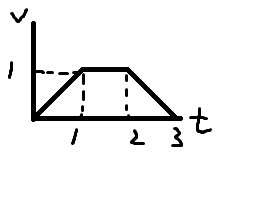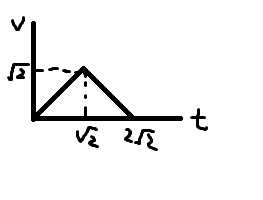# Elevator

Welcome back to OBCYSIO! Orange Boy has been promoted to LGM boy so this series is in an awkward situation.

This problem is very easy.

Consider an elevator with a max speed of v_m m/s and an acceleration of a m/s^2. When the elevator is moving, it accelerates to the max speed(if possible) then deaccelerates and reaches the destination the moment its speed drops to 0.

For example, when the distance to destination is 2m, a=1,v_m=1, the following v-t graph can be drawn and the time needed to reach the destination is 3s:When the distance to destination is 2m, a=1,v_m=2, the following v-t graph can be drawn and the time needed to reach the destination is 2\sqrt2s:There are n floors in the building, numbered from 1 to n. The height of each floor is h m. Now the elevator is at floor 1 and someone needs to get to floor n.

There are q queries, each query will either:

• Instruct the elevator to stop at floor x
• Cancel a previous instruction to stop at floor x

Note that the elevator does not actually move after each query.(ie They are imaginary queries)

(2\leq x\leq n-1)

What's the time needed to reach floor n after each query?

# Example

v_m=1,a=1,h=2,n=4
- With no queries: t=7s
- Add: stop at floor 2:t=3+5=8s
- Add: stop at floor 3:t=3+3+3=9s
- Remove: stop at floor 3:t=3+5=8s

# Limits

• given: 1\leq n,h,v_m,a\leq 10^9
• given: 1\leq q\leq 10^5

THE END